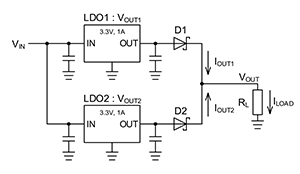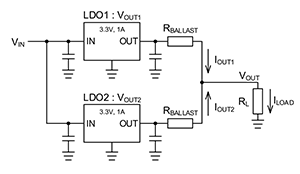Technical Information Site of Power Supply Design

2021.02.24 DC/DC

# Summary

Parallel Connections of LDO Linear Regulators

This article concludes the section on "Parallel Connections of LDO Linear Regulators." In this series of articles, we have explained methods for connecting LDOs in parallel to increase the output current of a power supply circuit and to avoid exceeding the allowable dissipation for individual LDOs.

As explained in ”About Parallel Connections of LDO Linear Regulators” parallel connection of LDOs is a method that has been around for quite some time. Basically and ideally, by for example connecting two 1 A LDOs in parallel, twice the current or 2 A is obtained, and from the standpoint of distribution of losses, when two devices connected in parallel the losses of each are halved.

However, if the outputs of LDOs are simply connected together directly, because there is a difference in the output voltages of the LDOs, the load (output) current is not distributed between them. In general, the tolerance in the output voltage of an LDO is stipulated to be within plus/minus a few percent of the nominal voltage, and as a practical matter, the output voltages of LDOs connected in parallel are never exactly the same. Hence a contrivance in the circuit is needed in order that the output current is distributed among the parallel-connected LDOs.

As parallel connection methods that distribute the output current, a method using diodes and a method using ballast resistors were presented. Both methods distribute the output current by relaxing the difference in the output voltages of the parallel-connected LDOs, but both have advantages and drawbacks. Moreover, it is difficult to perfectly realize "double/half" operation as per principles, and these methods must be used with the understanding that compromises are inevitable, given the output voltage precision, load regulation, and other power supply characteristics.

Below, the circuits of each of the parallel connection methods, equations representing the principles involved, and important points are presented.

Parallel Connection Using Diodes Parallel Connection Using Ballast ResistorsRelations for Balancing of Output Voltages

VOUT1-VF1(IOUT1)=VOUT2-VF(IOUT2)

VOUT1：LDO1 output voltage
VOUT2：LDO2 output voltage
VF1（IOUT1）： VF of D1 when current is IOUT1
VF2（IOUT2）： VF of D2 when current is IOUT2

VOUT1-IOUT1×RBALLAST=VOUT2-IOUT2×RBALLAST

VOUT1：LDO1 output voltage
VOUT2：LDO2 output voltage
IOUT1：LDO1 output current
IOUT2：LDO2 output current
RBALLAST：Ballast resistance

Key Points
• ・In parallel connections using diodes, because diodes are present in the output paths, the output voltages drop by the amount of the diode VF values, and so measures such as adding a voltage equivalent to VF to the LDO setting output voltage are necessary.
• ・There is scattering in diode VF values among devices, and the values also change according to the load current and the temperature, and therefore precision in the output voltage (load regulation) cannot be expected.
• ・Some buffering is achieved by inserting diodes, but in any case, the difference in the output voltages of two LDOs will cause considerable variation in the fractional output currents of the two LDOs.
• ・Parallel connections using ballast resistors can cause output currents to be distributed according to a value calculated using an equation.
• ・Output currents flow in the ballast resistors, so that a voltage drop occurs at the load point, and power losses occur in the resistors.
• ・There is a tradeoff between the difference in the LDO output voltages, the output current distribution, and the output voltage drop. The value of the ballast resistors must be determined while balancing all characteristics.